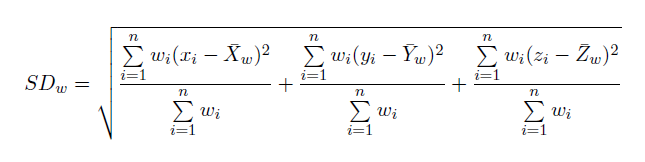# How Standard Distance works

Measuring the compactness of a distribution provides a single value representing the dispersion of features around the center. The value is a distance, so the compactness of a set of features can be represented on a map by drawing a circle or a sphere with the radius equal to the standard distance value. The Standard Distance tool creates a circle polygon or a multipatch sphere if your data is z enabled.

## Calculations

The Standard Distance is given as:

Where x i , y i and z i are the coordinates for feature i , {x̄, ȳ, z̄} represents the Mean Center for the features, and n is equal to the total number of features.

The Weighted Standard Distance extends to the following:Where wi is the weight at feature i and {x w, y w, z w} represents the weighted Mean Center.

## Output

For two-dimensional data, the Standard Distance tool creates a new feature class containing a circle polygon centered on the mean center (one center and one circle per case, if a Case Field is specified). Each circle polygon is drawn with a radius equal to the standard distance value. Attribute values for each circle polygon are the circle mean center x-coordinate, mean center y-coordinate, and standard distance (circle radius).

For three-dimensional data, the Standard Distance tool creates a new feature class containing a multipatch sphere centered on the mean center (one center and one sphere per case, if a Case Field is specified). Each multipatch sphere is drawn with a radius equal to the standard distance value. Attribute values for each multipatch sphere are the mean center x-coordinate, mean center y-coordinate, mean center z-coordinate, the sphere area, the volume of the sphere, and standard distance (sphere radius).

## Potential applications

• You can use the values for two or more distributions to compare them. A crime analyst, for example, could compare the compactness of assaults and auto thefts. Knowing how the different types of crimes are distributed may help police develop strategies for addressing the crime. If the distribution of crimes in a particular area is compact, stationing a single car near the center of the area might suffice. If the distribution is dispersed, having several police cars patrol the area might be more effective in responding to the crimes.
• You can also compare the same type of feature over different time periods—for example, a crime analyst could compare daytime and nighttime burglaries to see if burglaries are more dispersed or more compact during the day than at night.
• You can also compare the distributions of features to stationary features. For example, you could measure the distribution of emergency calls over several months for each responding fire station in a region and compare them to see which stations respond over a wider area.